Download Presentation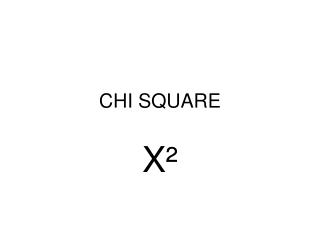CHI SQUARE

# CHI SQUARE - PowerPoint PPT Presentation

CHI SQUARE. X ². “MATHEMATICAL JUDGE” OF PROBABILITIES X ² = ∑{(O-E)²/E} ∑= the sum of O= observed E= expected. Chi square is used to measure the significance of the data in comparison with what you expect to get. Chi square table. Problem #1.I am the owner, or an agent authorized to act on behalf of the owner, of the copyrighted work described.
Download Presentation## CHI SQUARE

An Image/Link below is provided (as is) to download presentation

Download Policy: Content on the Website is provided to you AS IS for your information and personal use and may not be sold / licensed / shared on other websites without getting consent from its author.While downloading, if for some reason you are not able to download a presentation, the publisher may have deleted the file from their server.

- - - - - - - - - - - - - - - - - - - - - - - - - - E N D - - - - - - - - - - - - - - - - - - - - - - - - - -
Presentation Transcript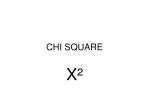### CHI SQUARE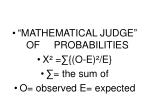“MATHEMATICAL JUDGE” OF PROBABILITIES
• X² =∑{(O-E)²/E}
• ∑= the sum of
• O= observed E= expected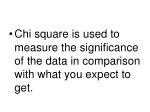Chi square is used to measure the significance of the data in comparison with what you expect to get.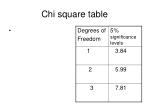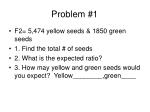Problem #1
• F2= 5,474 yellow seeds & 1850 green seeds
• 1. Find the total # of seeds
• 2. What is the expected ratio?
• 3. How may yellow and green seeds would you expect? Yellow________,green____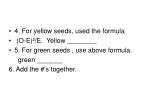4. For yellow seeds, used the formula
• (O-E)²/E. Yellow ________
• 5. For green seeds , use above formula.

green _______

6. Add the #’s together.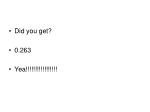Did you get?
• 0.263
• Yea!!!!!!!!!!!!!!!!!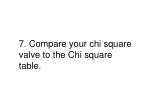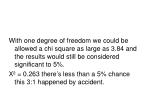With one degree of freedom we could be allowed a chi square as large as 3.84 and the results would still be considered significant to 5%.

X² = 0.263 there’s less than a 5% chance this 3:1 happened by accident.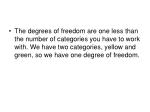The degrees of freedom are one less than the number of categories you have to work with. We have two categories, yellow and green, so we have one degree of freedom.Find the chi square for the C-fern.
• Use the class totals.
• The p= cc X Cc
• cc= polka dot and is recessive
• Cc = wild type and heterozygous
• What would the expected ratio for F1 be with this cross?